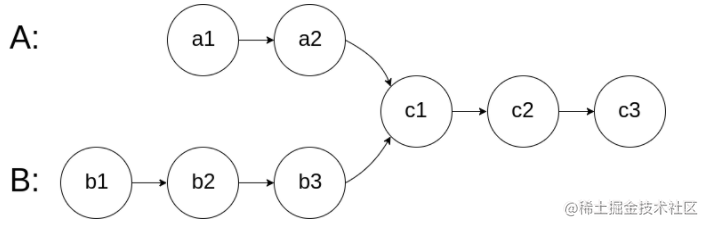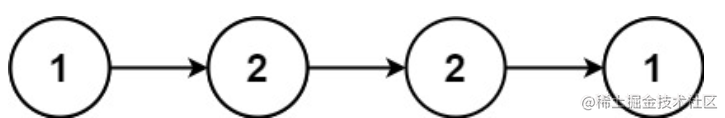探索数据结构小紫书之线性表

基本概念

• 线性表的链式存储结构的特点是用一组任意的存储单元存储线性表的数据元素（这组存储单元可以是连续的，也可以是不连续的）

• 一个结点，包含两个域，数据域和指针域

• n个结点链结成一个链表，即为线性表

各种操作

反转链表

/**
* @return {ListNode}
*/
//以下一个结点作为头结点
}
//递归
};

//迭代！ 双指针
let prev = null;//前指针
while (curr) {
const next = curr.next;//当前指针的下一个 存起来
curr.next = prev;
prev = curr;//前进
curr = next;//前进
}
return prev;
};

环形链表

//给每个节点打标记
return true;
}
}
return false;
};

链表中倒数第k个节点

var getKthFromEnd = function(head, k) {
//p慢指针，q快指针，q先走k步，然后p q一起走 当q节点为空，则返回p
while(q){
if(i>=k){
p=p.next
}
q=q.next
i++
}
return i>=k ? p : null
};

合并两个有序链表

var mergeTwoLists = function(l1, l2) {

while(l1!=null && l2!=null){//当有一个或两个都到头了跳出while
if(l1.val<=l2.val){
prev.next=l1;//当l1更小，那么指向l1
l1=l1.next//l1变为 l1.next  迭代
}else{
prev.next=l2//同理
l2=l2.next//同理
}
prev=prev.next//prev像后移一位
}
//合并到l1或者l2到头，判断谁还有，就把谁接上
prev.next = l1 === null?l2:l1
};

相交链表//相交链表，用哈希表解决
const visited = new Set();//Set结构  只存key 且key不重复
while(temp!==null){
temp=temp.next
}
while(temp!==null){
if(visited.has(temp)){
return temp
}
}
return null;//没找到就返回null
};

return null
}//有任何一个是空 则没有交点
while(pA!==pB){//当没找到相交点的时候
pA= pA===null ? headB : pA.next//没有找到就换个链表找
pB= pB===null ? headA : pB.next//同上
}//总是有人会先交换，有人后交换
//（除非两个链表长度一样，如果这样第一次就可以找到，不用交换）
//因为有这个先后，所以迟早会遇到走一样路程就可以到相交点的情况
return pA
};

回文链表

示例 1：//回文是指前后读起来都一个样子
//先存为数组 然后用双指针
const arr=[]//创建一个数组保存 链表的值
while(current){
arr.push(current.val)
current=current.next
}
let p,q;//双指针
for(let i=0;i<arr.length;i++){
p=arr[i]
q=arr[arr.length-1-i]
if(p!=q){//当遇到他们不一样时 则不对称
return false
}
}
return true
};